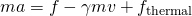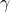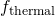The Markov model, without question, is one of the most powerful and elegant tools available in many fields of biological modeling and beyond.  In my world of molecular simulation, Markov models have provided analyses more insightful than would be possible with direct simulation alone.  And I’m a user, too.  Markov models, in their chemical-kinetics guise, play a prominent role in illustrating cellular biophysics in my online book, Physical Lens on the Cell.

Yet it’s fair to say that everything is Markovian and nothing is Markovian – and we need to understand this.

If you’re new to the business, a quick word on what “Markovian” means.  A Markov process is a stochastic process where the future (i.e., the distribution of future outcomes) depends only on the present state of the system.  Good examples would be chemical kinetics models with transition probabilities governed by rate constants or simple Monte Carlo simulation (a.k.a. Markov-chain Monte Carlo).  To determine the next state of the system, we don’t care about the past: only the present state matters.

What about systems following deterministic dynamics, e.g., following classical equations of motion, Newton’s laws?  Let’s say that such systems are “effectively Markovian” in that the future depends only on the present state of the system.  In such cases, we must include the full “phase space” of the system in the present state – i.e., velocities as well as positions of particles.

Back to those contradictory claims …

Everything is Markovian.  Really all the physics (and chemistry and biology) most of us are interested in, in fact, is Markovian or effectively so.  Once the states and velocities/momenta of all particles are accounted for, the future (or distribution of future outcomes) is determined.  This is not to say that you personally know how to figure out the distribution – only that the distribution is determined.  This is true for classical and quantum systems, stochastic and deterministic dynamics, you name it.  The only trick is to be sure your definition of the present state includes all necessary information.

Nothing is Markovian.  And yet, as you may know, for complex systems it is extremely challenging to build Markov models.  For that matter, why are they called “models” anyway if the true dynamics are (effectively) Markovian as just claimed above?  The answer is arguably in the eye of the beholder, or more precisely, in the subset of a system’s phase space chosen for analysis.  If only a subset of system coordinates is examined, or even if a discretized representation of the full set of coordinates is used, a systems behavior generally will appear non-Markovian.  In such a sub-space, we will not be able to predict the distribution of future outcomes knowing only the present, because we are ignoring system details that matter in the fundamental (effectively Markovian) dynamics.The idea that dynamics in sub-spaces appears to be non-Markovian is an old one.  The most famous example I know of arises in Langevin dynamics.  Standard Langevin dynamics is a Markovian process in the full phase space of all system configurational/positional coordinates and velocities: the future depends only on the present.  We can see this in the one-dimensional Langevin equation:whereis the “frictional frequency” opposing motion andthe random force due to thermal collisions; other symbols are standard.  If we specify the present/initial position and velocity, we specify the future distribution of outcomes.  However, if we examine a standard Langevin trajectory in only positional space, the behavior will appear non-Markovian: the future will depend not only on the present but also on the past – i.e., on inertial effects not represented in instantaneous positional coordinates.  In other words, to model the effect of inertia without velocities, one requires some previous history of the system to implicitly represent the velocity.Sometimes non-Markov effects can appear in subtle ways.  Imagine a simple two dimensional system, such as in the sketch, where our primary interest is in transitions in the x coordinate, from low to high values of x.  Assume that the true dynamics are Markovian in x and y, so no inertia to worry about in this abstract example.  Because we are only interested in transitions in x, it is natural to build a Markov model by binning in (subdividing) x and estimating transition rate among the bins.  Such a model will become more accurate as the bins become smaller.  But interestingly, for this energy landscape, Markovian behavior will never be recovered so long as the y coordinate is not also subdivided.  Why?  Because the probability to move left or right clearly will depend on whether the system is in the upper or lower energy valley: the upper one slopes downward to the right and the lower one slopes to the left.  As this example suggests, to guarantee recovery of Markovian behavior, bins must become uniformly small in all coordinates.The (apparently) non-Markovian behavior doesn’t emerge only in higher dimensional systems.  Consider simple diffusion in one dimension, as exemplified in the x vs. t trajectory shown.  Inspection of the trajectory shows that the transition probability from state 3 to 4 depends on the fact that the trajectory was previously in state 2.  Because the trajectory is strictly diffusive and Markovian in x space, when it reaches the boundary of states 2 and 3, it is equally likely to return to 2 … but less likely to get to 4 due to the finite time required to transit across bin 3.

What does this mean for “real” systems of interest?  The challenge in typical complex systems is that one has only a finite amount of trajectory data, and so making small bins – especially ones that are small in all dimensions – is effectively impossible.  There will not be enough transition counts among the bins to enable accurate estimation of the transition rates.  Finite states will be intrinsically non-Markovian.

Bottom line: In a finite discrete model of a complex system, you should expect the behavior to be non-Markovian.  It is entirely a separate question – and a topic of current research (see references) – whether a Markov approximation to the non-Markovian behavior is sufficiently accurate.